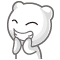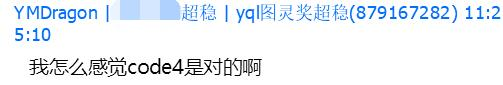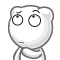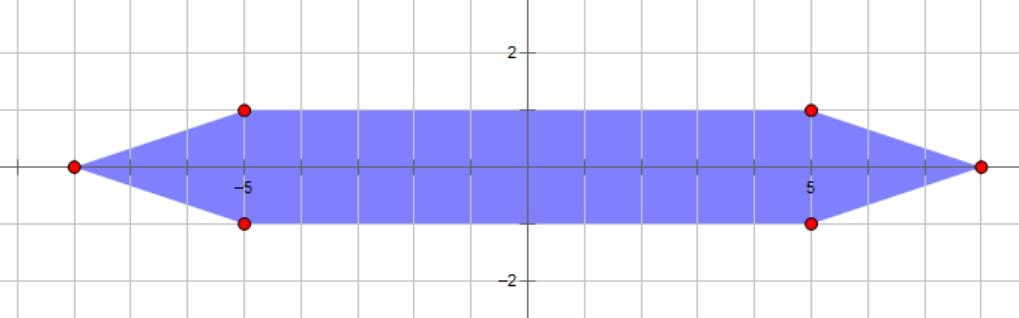# 博客

## UOJ NOI Round #3 Day2 题解

2018-07-14 15:46:20 By WuHongxun

### 白鸽

from matthew99， 题解 by matthew99

### 百鸽笼

from ztr， 题解 by ztr

### 鸽举选仕

from whzzt， 题解 by whzzt 首先作为出题人给大家道个歉……准备时间有点紧张，没有来得及对每个子任务进行认真的检查和加强，对UOJ系统不熟悉、赛中又出了若干口大小不等的锅，实在是有点难受啊……都怪*鸽普太鸽了(甩锅)

#### 子任务一

#include <stdio.h>
int a, b;
int ADD(int a, int b){ return (a ^ b) | (a & b) << 1; }
int main(){
scanf("%d%d", &a, &b);
printf("%d\n", ADD(a ^ b, (a & b) << 1));
return 0;
}

#### 子任务二

#include<stdio.h>
int main(){
int i;
puts("5000 10000");
for(i=2;i<=1000;i++)printf("1 1 %d\n",i);
puts("1 1000 5000");
for(i=4999;i>=1001;i--)printf("1 %d %d\n",i+1,i);
for(i=1;i<=5001;i++)printf("2 %d %d\n",i%1000+1,1001+i/1000);
}

#### 子任务三#include<bits/stdc++.h>
using namespace std;
int rnd(){return rand()<<15|rand();}
int rnd(int l,int r){return rnd()%(r-l+1)+l;}
const int N=100005,inf=2e9;
int n=100000,m,p[N],fa[N],fd1[N],fd2[N];
int main(){
int i;
for(i=1;i<=n;i++)p[i]=i;
for(i=1;i<=n;i++)swap(p[i],p[rnd(i,n)]);
m=n-100;
for(i=2;i<=m;i++)fa[i]=rnd(1,i-1),fd1[i]=rnd(-10,10),fd2[i]=rnd(-10,10);
fa[m+1]=1;fd1[m+1]=-inf;fd2[m+1]=-inf;
fa[m+2]=m+1;fd1[m+2]=inf;fd2[m+2]=rnd(1,10);
fa[m+3]=m+1;fd1[m+3]=inf;fd2[m+3]=rnd(1,10);
fa[m+4]=m;fd1[m+4]=-inf;fd2[m+4]=-inf;
for(i=m+5;i<=n;i++)fa[i]=i-1,fd1[i]=-inf+rnd(-10,10),fd2[i]=-inf+rnd(-10,10);
//best solution: m+2, m+3
printf("%d\n",n);
for(i=2;i<=n;i++)printf("%d %d %d\n",p[fa[i]],p[i],fd1[i]);
for(i=2;i<=n;i++)printf("%d %d %d\n",p[fa[i]],p[i],fd2[i]);
}

#### 子任务四12 4
0 0 0 70 80 90 1000 1000 1000 200 180 199

#### 子任务五

#include<bits/stdc++.h>
using namespace std;
const int N=100005,B=1e7,Q=1e8,R=Q-1,inf=1e9;
int n=100000,m=99990,l[N],r[N];
int main(){
int i;
for(i=1;i<=m;i++)l[i]=i,r[i]=i+R;
l[n]=inf-Q;r[n]=inf;l[n-1]=inf-Q-1;r[n-1]=inf-1;
for(i=m+1;i<=n-2;i++)l[i]=l[n]-B*(n-i),r[i]=l[n]+i;
printf("%d\n",n);
for(i=1;i<=n;i++)printf("%d %d\n",l[i],r[i]);
return 0;
}

#### 子任务六## 评论

2000F11Y10M123
!前排~
peehs_moorhsum

Magolor
code7一股@OldDriverTree的气息
tlyangwj

Marco_L_Tsien

wangxiuhan

oscar

tianfuzhen

applese
T3的第二个点是不是还可以卡wa啊.. while(q--)的时候在bfs里vis[a]=q了， 只要最后一个操作是询问他好像一定输出0的样子啊。。 我是这样过的..
WildRage

yanQval

star_magic_young

mcfxmcfx

wzf2000
T2 算法七应该是由算法六优化的吧。怕是算法五是后来加上的。
kczno1
C题第四个的转移中dp[max(i - m, 0)] + a[q[head]]中的a[q[head]]是 $a_{i-m},a_{i-m+1},\cdots,a_{i}$ 中的最大值，而不是 $a_{i-m+1},\cdots,a_i$ 中的最大值，所依可以通过这个来卡。